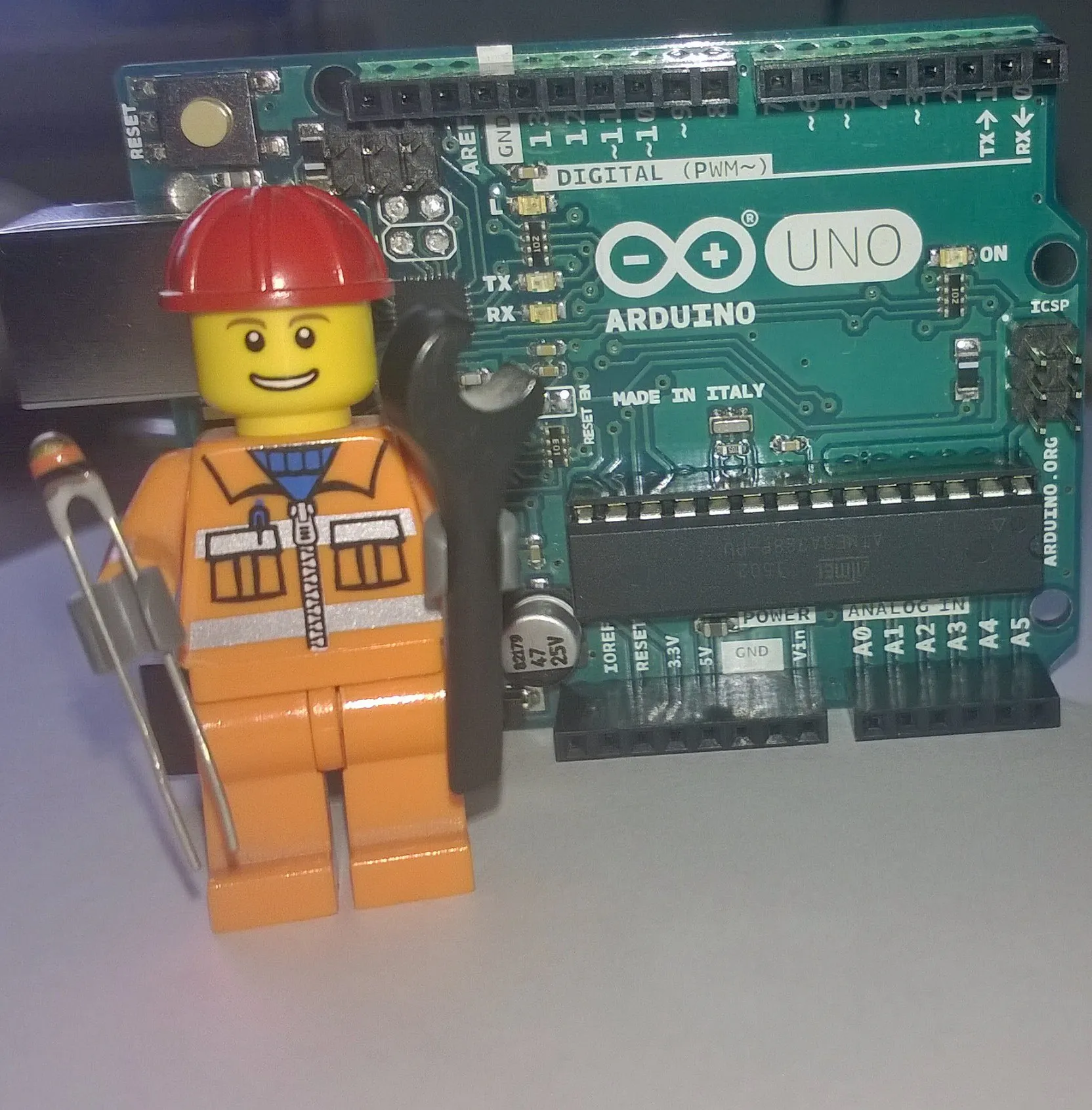Project tutorialHow Easy Is It to Use a Thermistor?! © GPL3+

Thermistor as thermometer.

• 9,149 views
• 13 respects

Apps and online services

How easy is it to use a thermistor?!

In this article I will explain how to use a thermistor. First of all, what is it a Thermistor? A thermistor is a type of resistor whose resistance is dependent on temperature. There are two opposite types of thermistor:

• PTC (Positve Temperature Coefficent), resistance increases as temperature rises
• NTC (Negative Temperature Coefficent), resistance decreases as temperature rises

In this case I use NTC.

A little bit of math.

To calculate the thermistor resistance using a simple formula called equation with parameter B (with only NTC termistor).

Where:

• e is the base of natural logarithm
• R0 is the resistance of the thermistor measured at the temperature T0
• B is a constant coefficient that depends on the characteristics of the material, it is a constant expressed in K, and its value is indicated by the manufacturers on the technical sheets

To calculate the temperature we need know the resistance RT using the Ohm's laws.

This is a schematic version of circuit.

RT = VRT / (VR/R)

Now we have all the data to calculate the temperature.

Remember to convert all parameters (for example T0) to Kelvin before the calculations, and also the result is in Kelvin.

Code

ThermistorArduino
//Thermometer with thermistor

/*thermistor parameters:
* RT0: 10 000 Ω
* B: 3977 K +- 0.75%
* T0:  25 C
* +- 5%
*/

//These values are in the datasheet
#define RT0 10000   // Ω
#define B 3977      // K
//--------------------------------------

#define VCC 5    //Supply voltage
#define R 10000  //R=10KΩ

//Variables
float RT, VR, ln, TX, T0, VRT;

void setup() {
Serial.begin(9600);
T0 = 25 + 273.15;                 //Temperature T0 from datasheet, conversion from Celsius to kelvin
}

void loop() {
VRT = analogRead(A0);              //Acquisition analog value of VRT
VRT = (5.00 / 1023.00) * VRT;      //Conversion to voltage
VR = VCC - VRT;
RT = VRT / (VR / R);               //Resistance of RT

ln = log(RT / RT0);
TX = (1 / ((ln / B) + (1 / T0))); //Temperature from thermistor

TX = TX - 273.15;                 //Conversion to Celsius

Serial.print("Temperature:");
Serial.print("\t");
Serial.print(TX);
Serial.print("C\t\t");
Serial.print(TX + 273.15);        //Conversion to Kelvin
Serial.print("K\t\t");
Serial.print((TX * 1.8) + 32);    //Conversion to Fahrenheit
Serial.println("F");
delay(500);

}

SchematicsUnravel Preset Piano Easy Arduino (Even a Ghoul Can Make It)

Project in progress by ExeCuteLi

• 5,487 views
• 20 respects

Easy Thermometer v1

Project tutorial by hixel

• 1,318 views
• 1 comment
• 3 respects

Arduino + Raspberry Pi + Ubidots Made Easy

Project tutorial by David Escobar

• 13,094 views
• 38 respects

Easy Countdown with Buzzer

Project showcase by Marcazzan_M

• 10,368 views
• 13 respects

Hackster Live April 2017 Workshop - Optional - Easy Add-on

Project tutorial by Katie Kristoff

• 883 views